Function Repository Resource:

# SmallestPartsFunction

Total number of smallest parts in the partitions of a positive integer

Contributed by: George Beck
 ResourceFunction["SmallestPartsFunction"][n] gives the total number of smallest parts in the integer partitions of the positive integer n.

## Details and Options

A partition of n is a list of weakly decreasing positive integers that add up to n. For instance, written compactly, these are the five partitions of 4: 4, 31, 22, 211, 1111.
ResourceFunction["SmallestPartsFunction"][n] returns the Total of all the minimal elements in all the partitions of n.

## Examples

### Basic Examples (2)

Here is an illustrative example. Start with the integer partitions of 3:

 In:=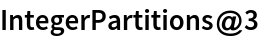Out=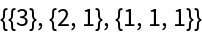Here are their smallest parts:

 In:=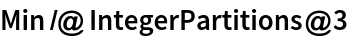Out=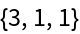Compute the number of smallest parts per partition:

 In:=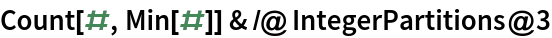Out=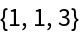Find their total:

 In:=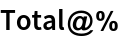Out=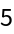This is precisely the value returned by SmallestPartsFunction:

 In:=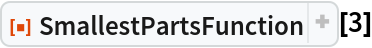Out=Here are the first 10 numbers in the smallest part function (denoted spt) sequence (OEIS A092269):

 In:=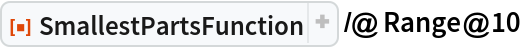Out=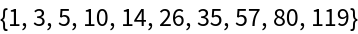### Neat Examples (3)

The function spt(n) satisfies some congruences similar to Ramanujan’s congruences for the partition function p(n).

Numbers of the form 5n+4 have spt divisible by 5:

 In:=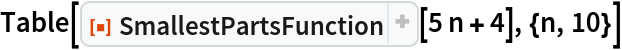Out=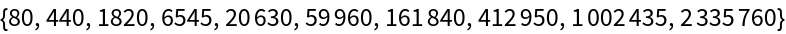In:=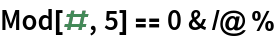Out=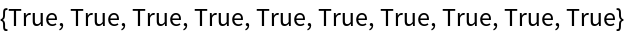Numbers of the form 7n+5 have spt divisible by 7:

 In:=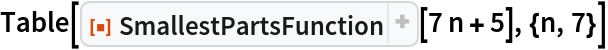Out=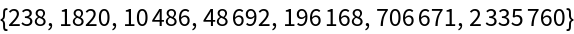In:=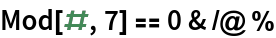Out=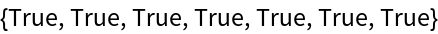Numbers of the form 13n+6 have spt divisible by 13:

 In:=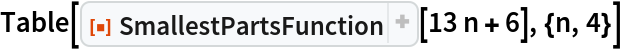Out=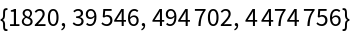In:=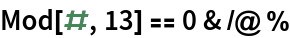Out=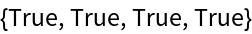George Beck

## Version History

• 1.0.0 – 31 May 2019